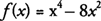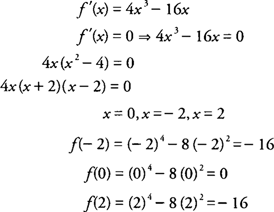## Critical Points

Points on the graph of a function where the derivative is zero or the derivative does not exist are important to consider in many application problems of the derivative. The point ( x, f(x)) is called a critical point of f(x) if x is in the domain of the function and either f′(x) = 0 or f′(x) does not exist. The geometric interpretation of what is taking place at a critical point is that the tangent line is either horizontal, vertical, or does not exist at that point on the curve.

Example 1: Find all critical points of.

Because f(x) is a polynomial function, its domain is all real numbers.hence, the critical points of f(x) are (−2,−16), (0,0), and (2,−16).

Example 2: Find all critical points of f(x)= sin x + cos x on [0,2π].

The domain of f(x) is restricted to the closed interval [0,2π].hence, the critical points of f(x) areandBack to Top
A18ACD436D5A3997E3DA2573E3FD792A